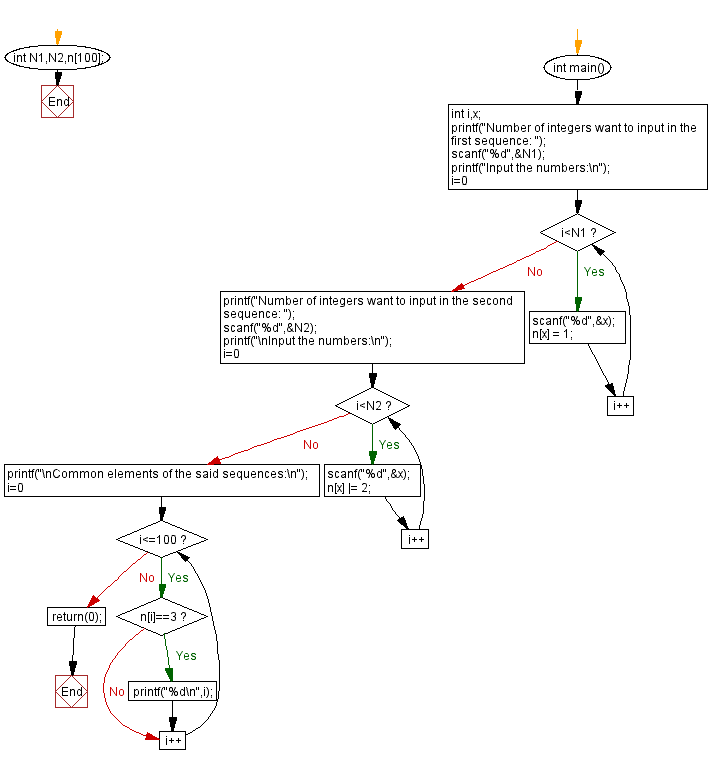﻿ C - Common element(s) of two sequences

# C Exercises: Common element(s) of two sequences

## C Basic-II: Exercise-4 with Solution

Write a C program that accepts two sequences ((a1, a2, .. an), (b1, b2, .. bn)) of integers as input. Find all integers that appear in both sequences, one by one, in ascending order.

Constraints:

• 1≤N1≤100, N1 -> number of integers in first sequence.
• 1≤N2≤100, N2 -> number of integers in second sequence.
• 1 ≤ ai≤ 100 ( 1 ≤ i ≤ N1 )
• 1 ≤ bj≤ 100 ( 1 ≤ j ≤ N2 )

Sample Date:

( 1 2 3 1 2 3 4) -> 1 2 3
( 1 2 3 1 2 3) -> 1 2 3
(1 2 3 4 5 6) ->

C Code:

``````#include <stdio.h> // Include standard input/output library

int N1, N2, n; // Declare global variables for sequence lengths and array 'n' to track occurrences

int main()
{
int i, x; // Declare local variables for iteration and input

// Prompt the user for the number of integers in the first sequence
printf("Number of integers want to input in the first sequence: ");

// Read the input and store it in 'N1'
scanf("%d", &N1);

// Prompt the user to input numbers for the first sequence
printf("Input the numbers:\n");

// Loop to read integers for the first sequence and mark them in 'n' array
for (i = 0; i< N1; i++)
{
// Read an integer from the user
scanf("%d", &x);

// Mark occurrence of the integer in 'n' array
n[x] = 1;
}

// Prompt the user for the number of integers in the second sequence
printf("Number of integers want to input in the second sequence: ");

// Read the input and store it in 'N2'
scanf("%d", &N2);

// Prompt the user to input numbers for the second sequence
printf("\nInput the numbers:\n");

// Loop to read integers for the second sequence and mark them in 'n' array
for (i = 0; i< N2; i++)
{
// Read an integer from the user
scanf("%d", &x);

// Mark occurrence of the integer in 'n' array (using bitwise OR operation)
n[x] |= 2;
}

// Print a newline for formatting
printf("\n");

// Print the common elements of the two sequences
printf("Common elements of the said sequences:\n");

// Loop to check 'n' array for elements marked as both 1 and 2 (binary 3)
for (i = 0; i<= 100; i++)
{
if (n[i] == 3)
printf("%d\n", i); // Print the common element
}

// Return 0 to indicate successful program execution
return 0;
}
``````

Sample Output:

```Number of integers want to input in the first sequence: 3
Input the numbers:
1 2 3
Number of integers want to input in the second sequence: 3

Input the numbers:
1 2 3

Common elements of the said sequences:
1
2
3
100
```

Flowchart:C Programming Code Editor:

Previous C Programming Exercise: Second largest among three integers.
Next C Programming Exercise: Sum values before, after the maximum value in a sequence.

What is the difficulty level of this exercise?

Test your Programming skills with w3resource's quiz.

﻿